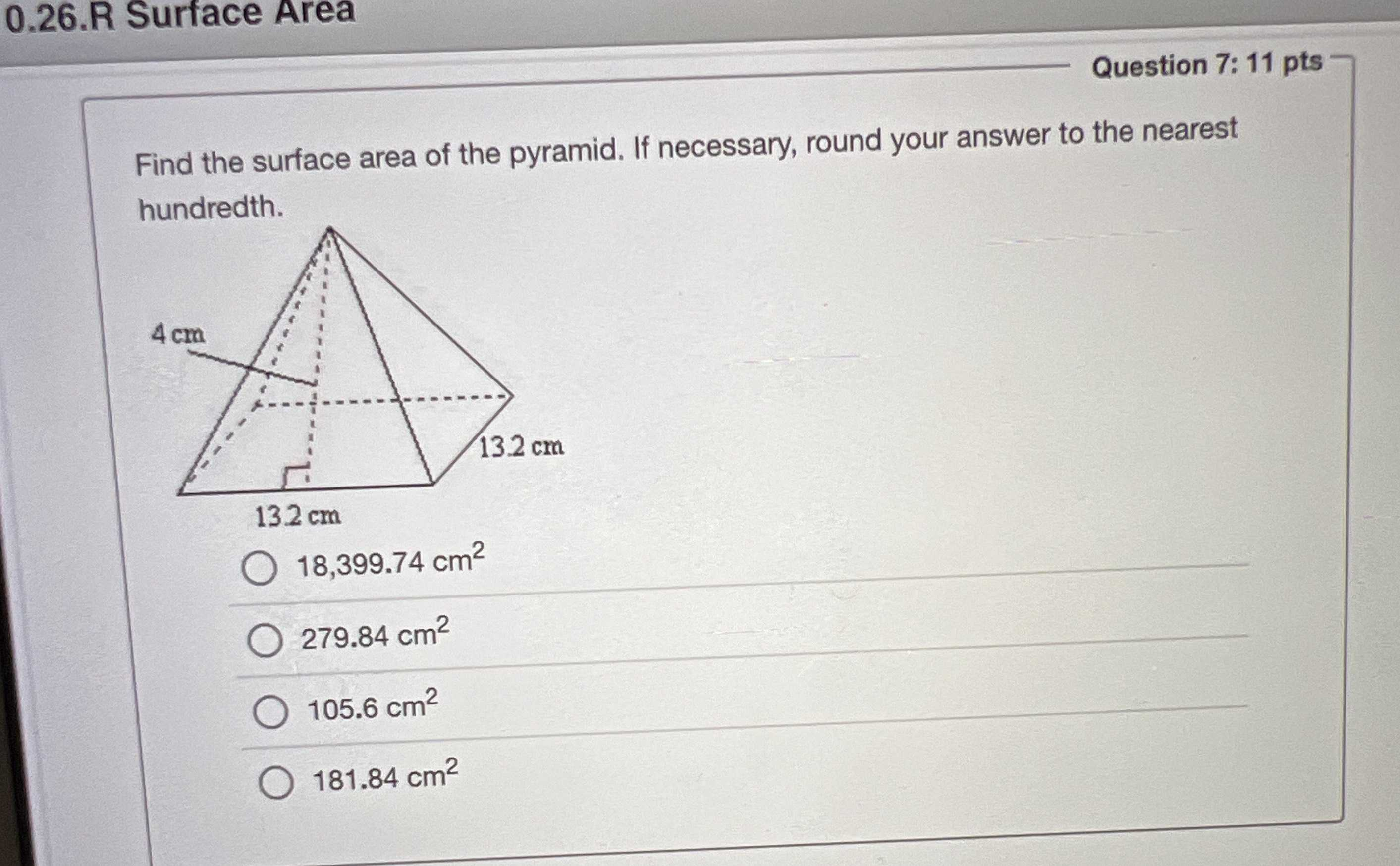### Still have math questions?

Trigonometry
QuestionFind the surface area of the pyramid. If necessary, round your answer to the nearest hundredth. $$18,399.74 cm ^ { 2 }$$

$$279.84 cm ^ { 2 }$$

$$105.6 cm ^ { 2 }$$

$$181.84 cm ^ { 2 }$$

B. 279.84 cm$$^2$$